# Principal Component Analysis (PCA) in PythonAn in-depth tutorial on principal component analysis (PCA) with mathematics and Python coding examples. Principal component analysis (PCA) is essential for data science, machine learning, data visualization, statistics, and other quantitative fields.

An in-depth tutorial on principal component analysis (PCA) with mathematics and Python coding examples

This tutorial’s code is available on Github and its full implementation as well on Google Colab.

1. Introduction
2. Curse of Dimensionality
3. Dimensionality Reduction
4. Correlation and its Measurement
5. Feature Selection
6. Feature Extraction
7. Linear Feature Extraction
8. Principal Component Analysis (PCA)
9. Math behind PCA
10. How does PCA work?
11. Applications of PCA
12. Implementation of PCA with Python
13. Conclusion

## Introduction

When implementing machine learning algorithms, the inclusion of more features might lead to worsening performance issues. Increasing the number of features will not always improve classification accuracy, which is also known as the curse of dimensionality. Hence, we apply dimensionality reduction to improve classification accuracy by selecting the optimal set of lower dimensionality features.

Principal component analysis (PCA) is essential for data science, machine learning, data visualization, statistics, and other quantitative fields.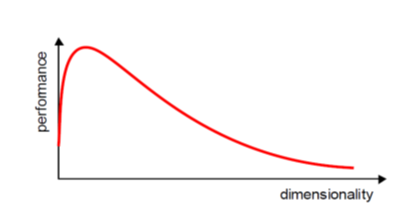Figure 1: Curse of dimensionality.

There are two techniques to make dimensionality reduction:

• Feature Selection
• Feature Extraction

It is essential to know about vector, matrix, and transpose matrix, eigenvalues, eigenvectors, and others to understand the concept of dimensionality reduction.

## Curse of Dimensionality

Dimensionality in a dataset becomes a severe impediment to achieve a reasonable efficiency for most algorithms. Increasing the number of features does not always improve accuracy. When data does not have enough features, the model is likely to underfit, and when data has too many features, it is likely to overfit. Hence it is called the curse of dimensionality. The curse of dimensionality is an astonishing paradox for data scientists, based on the exploding amount of n-dimensional spaces — as the number of dimensions, n, increases.

### Sparseness

The sparseness of data is the property of being scanty or scattered. It lacks denseness, and its high percentage of the variable’s cells do not contain actual data. Fundamentally full of “empty” or “N/A” values.

Points in an n-dimensional space frequently become sparse as the number of dimensions grows. The distance between points will extend to grow as the number of dimensions increases.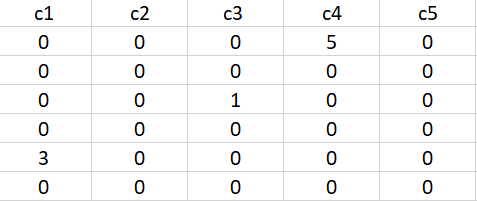Figure 2: Data sparseness.

### Implications of the Curse of Dimensionality

There are few implications of the curse of dimensionality:

• Optimization problems will be infeasible as the number of features increases.
• Due to the absolute scale of inherent points in an n-dimensional space, as n maintains to grow, the possibility of recognizing a particular point (or even a nearby point) proceeds to fall.

## Dimensionality Reduction

Dimensionality reduction eliminates some features of the dataset and creates a restricted set of features that contains all of the information needed to predict the target variables more efficiently and accurately.

Reducing the number of features normally also reduces the output variability and complexity of the learning process. The covariance matrix is an important step in the dimensionality reduction process. It is a critical process to check the correlation between different features.

## Correlation and its Measurement

There is a concept of correlation in machine learning that is called multicollinearity. Multicollinearity exists when one or more independent variables highly correlate with each other. Multicollinearity makes variables highly correlated to one another, which makes the variables’ coefficients highly unstable .

The coefficient is a significant part of regression, and if this is unstable, then there will be a poor outcome of the regression result. Multicollinearity is confirmed by using Variance Inflation Factors (VIF). Therefore, if multicollinearity is suspected, it can be checked using the variance inflation factor (VIF).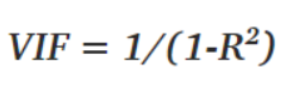Figure 3: VIF equation.

Rules from VIF:

• A VIF of 1 would indicate complete independence from any other variable.
• A VIF between 5 and 10 indicates a very high level of collinearity .
• The closer we get to 1, the more ideal the scenario for predictive modeling.
• Each independent variable regresses against each independent variable, and we calculate the VIF.

Heatmap also plays a crucial role in understanding the correlation between variables.

The type of relationship between any two quantities varies over a period of time.

Correlation varies from -1 *to *+1

To be precise,

• Values that are close to +1 indicate a positive correlation.
• Values close to -1 indicate a negative correlation.
• Values close to 0 indicate no correlation at all.

Below is the heatmap to show how we will correlate which features are highly dependent on the target feature and consider them.

### The Covariance Matrix and Heatmap

The covariance matrix is the first step in dimensionality reduction because it gives an idea of the number of features that strongly relate, and it is usually the first step in dimensionality reduction because it gives an idea of the number of strongly related features so that those features can be discarded.

It also gives the detail of all independent features. It provides an idea of the correlation between all the different pairs of features.

Identification of features in Iris dataset that are strongly correlated

Import all the required packages:

``````import numpy as np
import pandas as pd
from sklearn import datasets
import matplotlib.pyplot as plt``````

``````iris = datasets.load_iris()
iris.data``````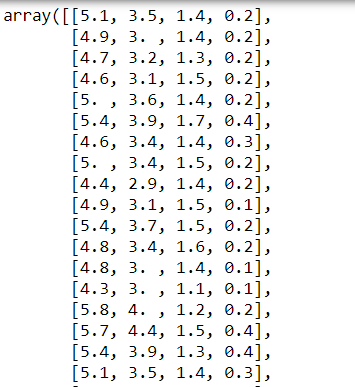Figure 4: Iris dataset.

List all features:

``iris.feature_names``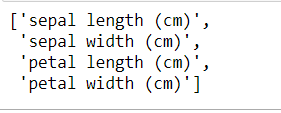Figure 5: Features of the Iris dataset.

Create a covariance matrix:

``cov_data = np.corrcoef(iris.data.T)cov_data``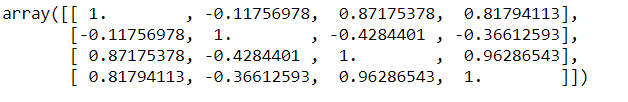Figure 6: Covariance matrix of the Iris dataset.

Plot the covariance matrix to identify the correlation between features using a heatmap:

``````img = plt.matshow(cov_data, cmap=plt.cm.rainbow)
plt.colorbar(img, ticks = [-1, 0, 1], fraction=0.045)for x in range(cov_data.shape):
for y in range(cov_data.shape):
plt.text(x, y, "%0.2f" % cov_data[x,y], size=12, color='black', ha="center", va="center")

plt.show()``````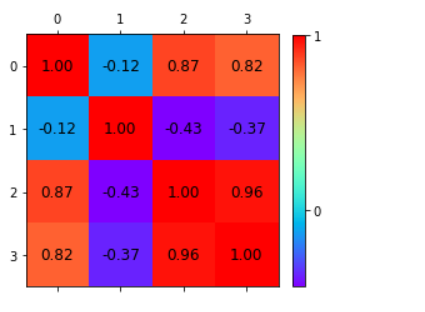Figure 7: Heatmap of the correlation matrix.

A correlation from the representation of the heatmap:

• Among the first and the third features.
• Between the first and the fourth features.
• Between the third and the fourth features.

Independent features:

• The second feature is almost independent of the others.

Here the correlation matrix and its pictorial representation have given the idea about the potential number of features reduction. Therefore, two features can be kept, and other features can be reduced apart from those two features.

There are two ways of dimensionality reduction:

• Feature Selection
• Feature Extraction

Dimensionality Reduction can ignore the components of lesser significance.

## Hire Machine Learning Developers in India

We supply you with world class machine learning experts / ML Developers with years of domain experience who can add more value to your business.

## Data Science Projects | Data Science | Machine Learning | Python

Practice your skills in Data Science with Python, by learning and then trying all these hands-on, interactive projects, that I have posted for you.

## Data Science Projects | Data Science | Machine Learning | Python

Practice your skills in Data Science with Python, by learning and then trying all these hands-on, interactive projects, that I have posted for you.

## Data Science Projects | Data Science | Machine Learning | Python

Practice your skills in Data Science with Python, by learning and then trying all these hands-on, interactive projects, that I have posted for you.

## Data Science Projects | Data Science | Machine Learning | Python

Practice your skills in Data Science with Python, by learning and then trying all these hands-on, interactive projects, that I have posted for you.